# GRE Subject Test: Chemistry : Freezing Point

## Example Questions

### Example Question #1 : Colligative Properties

What is the freezing point of a 2M solution of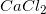in water?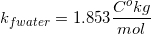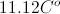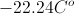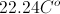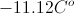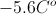Explanation: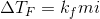First, we need to calculate the molality because that is what we use in our equation for freezing point depression. We can get that from the molarity without knowing exactly how many liters or grams we have. We just have to know what we have one mole per liter. The weight of water is one kilogram per liter, so this allows us to make this conversion.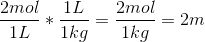The molality is 2m. The van't Hoff factor is 3, as we get one calcium ion and two chloride ions per molecule during dissociation.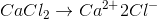We can now plug the values into the equation for freezing point depression.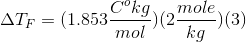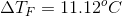This gives us our depression of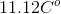.  The normal freezing point of pure water is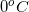, which means our new freezing point is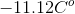.

### Example Question #1 : Physical Chemistry

How much sodium chloride has been added to four liters of water if the freezing point of the solution is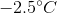?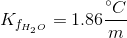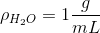Sodium chloride has a molar mass of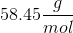.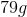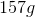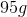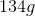Explanation:

We can determine how much sodium chloride was added to the water using the freezing point depression equation.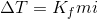The normal freezing point of wtaer is 0 degrees Celsius, so we know that the temperature of the solution has changed by 2.5 degrees. Since sodium chloride will generate two ions per molecule in solution, the van't Hoff factor will be 2. Based on the density of water, we can determine that 4 liters of water weighs 4 kilograms.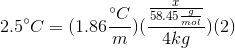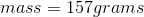### All GRE Subject Test: Chemistry Resources